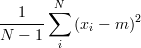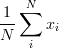# Problem Set 1: An introduction to OCaml

### Instructions

This problem set has three parts. You will write your solution to each part in a separate file: part1.txt, part2.ml, and part3.ml. To get you started, we have provided a stub file for each part. You should download and edit these files. Once you have finished, submit your solution using CMS, linked from the course website.

1. Compile errors: All programs that you submit must compile. Programs that do not compile will probably receive an automatic zero. If you are having trouble getting your assignment to compile, please visit consulting hours. If you run out of time, it is better to comment out the parts that do not compile and hand in a file that compiles, rather than handing in a more complete file that does not compile.
2. Missing functions: We will be using an automatic grading script, so it is crucial that you name your functions and order their arguments according to the problem set instructions, and that you place the functions in the correct files. Otherwise you may not receive credit for a function properly written.
3. Code style: Finally, please pay attention to style. Refer to the CS 3110 style guide and lecture notes. Ugly code that is functionally correct may still lose points. Take the extra time to think out the problems and find the most elegant solutions before coding them up. Even though only the second part of the assignment explicitly addresses the style issue, good programming style is also required for the other parts of this assignment, and for all the subsequent assignments in the rest of the semester.

### Part 1: Expression Types

Give the types of each of the following OCaml expressions. For example, the type of (1, 2) is int * int.

1. [("cs", 3110); ("cs", 312)]
2. (fun (x, y) -> x *. y) (4.0, 5.0)
3. (Some 123, None)

Give expressions that have the following OCaml types:

1. ((int * float) list * string) list
2. (float -> float -> float) * (int * int -> int)

Replace ??? with an expression that makes the code type check correctly. Give the type of the expression you chose.

1. let rec zardoz foo =
match foo with
((a, b), c)::xs -> a (b + c + (zardoz xs))
| _ -> 1
in zardoz ???

2. let vector = (32.0, 28.0) in
let sq x = x *. x in
let magnitude x y = sqrt(sq x +. sq y) in
let uncurry = ??? in
let zardoz = int_of_float (uncurry magnitude vector) in zardoz


### Part 2: Code Style

The following function executes correctly, but it was written with poor style. Your task is to rewrite it with better style. Please consult the CS 3110 style guide. You may not use the standard library function List.merge for this problem. Make sure that you use pattern matching.

Correction on 9/7: The code for this function was changed to correct a bug: merge []  should return .
let rec merge a b =
if (List.length a) = 0 && (List.length b) = 0 then b
else if (List.length b) = 0 then a
else if (List.length a) = 0 then b
else if (List.hd(a) < List.hd(b)) = true then [List.hd a]
@ (merge (List.tl a) b) else [List.hd b] @ (merge a (List.tl b))


### Part 3: OCaml Functinons

1. Write a function sorted : int list -> bool that returns true if the items in the list are in non-descending order. The empty list is considered to be sorted. For efficiency reasons, try not to sort a copy of the list and compare it to the original.

For example, sorted [1; 1; 2; 4] = true and sorted [1; 2; 3; 1] = false

2. Write a function perfect : int -> bool that returns true if its input is a perfect number. A perfect number is a positive integer that is equal to the sum of its positive divisors except itself.

For example, perfect 6 = true and perfect 100 = false

3. The type intlist can represent an int list.

type intlist = Nil | Cons of int * intlist


For example, Nil represents the empty list and Cons(1, Cons(2, Cons(3, Nil))) represents [1; 2; 3]

 Write a function partition : intlist -> int -> intlist * intlist that takes an intlist and returns a tuple (less, greater) where less is an intlist that contains all of the values from lst that are less than or equal to n, and greater contains all of the values greater than n. If a value appears multiple times in lst then it should appear the same number of times in one of the returned intlists. 
4.  
5.  Write a function variance : float list -> float that returns the sample variance of the numbers in its input list. Sample variance is defined to bewhere xi is the ith value of the input list, N is the number of values in the input list, and m is the arithmetic mean:. For example, variance [1.0; 2.0; 3.0; 4.0; 5.0] = 2.5. Remember to use the floating point version of the arithmetic operators when operating on floats (i.e. use +., *., and /. instead of +, *, and /). Use the float function to cast in int to a float.
6. Write a function permutations : int list -> int list list such that permutations lst returns a list containing every permutation of lst. For example, one correct answer to permutations [1; 2; 3] is [[1; 2; 3]; [2; 1; 3]; [2; 3; 1]; [1; 3; 2]; [3; 1; 2]; [3; 2; 1]]. It doesn't matter what order the permutations appear in the returned list. Note that if the input list is of length n then the answer should be of length n!.